Dr. S. R. Lasker Library Online Catalogue

Normal view

# A first course in Bayesian statistical methods / Peter D. Hoff.

Material type:TextLanguage: English Series: Springer texts in statisticsPublication details: c2009. Description: ix, 270 p. : ill. ; 24 cmISBN: 9780387922997 (hbk. : acidfree paper); 0387922997 (hbk.); 9780387924076 (eISBN); 0387924078DDC classification: 519.5 LOC classification: QA279.5 | .H64 2009Online resources: Table of contents | WorldCat details | E-book Fulltext
Contents:
TOC Introduction and examples -- belief, probability and exchangeability -- One-parameter models -- Monte Carlo approximation -- the normal model -- Posterior approximation with the Gibbs sampler -- the multivariate normal model -- Group comparisons and hierarchical modeling -- Linear regression -- Nonconjugate priors and Metropolis-Hastings algorithms -- Linear and generalized linear mixed effects models -- Latent variable methods for ordinal data.
Summary: This compact, self-contained introduction to the theory and application of Bayesian statistical methods is accessible to those with a basic familiarity with probability, yet allows advanced readers to grasp the principles underlying Bayesian theory and method.
Tags from this library: No tags from this library for this title.
Star ratingsAverage rating: 0.0 (0 votes)
Holdings
Item type Current library Collection Call number Copy number Status Date due Barcode Item holdsE-Book
E-book
Non-fiction 519.5 HOF 2009 (Browse shelf(Opens below)) Not for loanText
Reserve Section
Non-fiction 519.5 HOF 2009 (Browse shelf(Opens below)) C-1 Not For Loan 26679
Total holds: 0
##### Browsing EWU Library shelves, Shelving location: Reserve Section Close shelf browser (Hides shelf browser)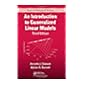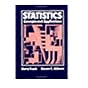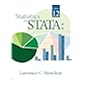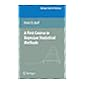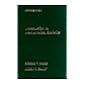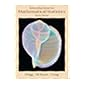519.5 DOI 2008 An introduction to generalized linear models / 519.5 FRS 1994 Statistics : 519.5 HAS 2013 Statistics with Stata : 519.5 HOF 2009 A first course in Bayesian statistical methods / 519.5 HOI 1995 Introduction to mathematical statistics / 519.5 HOI 2005 Introduction to mathematical statistics / 519.5 HOI 2005 Introduction to mathematical statistics /

Includes bibliographical references (p. -265) and index.

TOC Introduction and examples -- belief, probability and exchangeability -- One-parameter models -- Monte Carlo approximation -- the normal model -- Posterior approximation with the Gibbs sampler -- the multivariate normal model -- Group comparisons and hierarchical modeling -- Linear regression -- Nonconjugate priors and Metropolis-Hastings algorithms -- Linear and generalized linear mixed effects models -- Latent variable methods for ordinal data.

This compact, self-contained introduction to the theory and application of Bayesian statistical methods is accessible to those with a basic familiarity with probability, yet allows advanced readers to grasp the principles underlying Bayesian theory and method.

AS

Tahur Ahmed

There are no comments on this title.